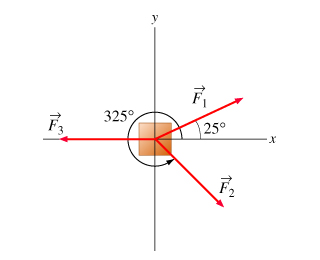# Problem: Three forces of magnitudes F1=4.0N, F2=6.0N, and F3=8.0N are applied to a block of mass m=2.0kg, initially at rest, at angles shown on the diagram. (Figure 1) In this problem, you will determine the resultant (net) force by combining the three individual force vectors. All angles should be measured counterclockwise from the positive x axis (i.e., all angles are positive).Part A: Calculate the magnitude of the resultant force acting on the block.Part B: What angle does Fr make with the positive x axis?

###### FREE Expert Solution

Force in a vector form:

$\overline{)\stackrel{\mathbf{⇀}}{\mathbf{F}}{\mathbf{=}}{\mathbf{F}}{\mathbf{c}}{\mathbf{o}}{\mathbf{s}}{\mathbf{\theta }}\stackrel{\mathbf{^}}{\mathbf{x}}{\mathbf{+}}{\mathbf{F}}{\mathbf{s}}{\mathbf{i}}{\mathbf{n}}{\mathbf{\theta }}\stackrel{\mathbf{^}}{\mathbf{y}}}$

Vector Magnitude:

$\overline{)\mathbf{|}\stackrel{\mathbf{⇀}}{\mathbit{A}}\mathbf{|}{\mathbf{=}}\sqrt{{{\mathbit{A}}_{\mathbit{x}}}^{\mathbf{2}}\mathbf{+}{{\mathbit{A}}_{\mathbit{y}}}^{\mathbf{2}}}}$

Direction:

$\overline{){\mathbf{tan}}{\mathbit{\theta }}{\mathbf{=}}\frac{{\mathbit{A}}_{\mathbit{y}}}{{\mathbit{A}}_{\mathbit{x}}}}$

The magnitude of the resultant force, Fr = F1 + F2 + F3

Part A

F1 = (4.0)cos(25°)x + (4.0)sin(25°)y = 3.63x + 1.69y

81% (91 ratings)###### Problem Details

Three forces of magnitudes F1=4.0N, F2=6.0N, and F3=8.0N are applied to a block of mass m=2.0kg, initially at rest, at angles shown on the diagram. (Figure 1) In this problem, you will determine the resultant (net) force by combining the three individual force vectors. All angles should be measured counterclockwise from the positive x axis (i.e., all angles are positive).Part A: Calculate the magnitude of the resultant force acting on the block.

Part B: What angle does Fr make with the positive x axis?